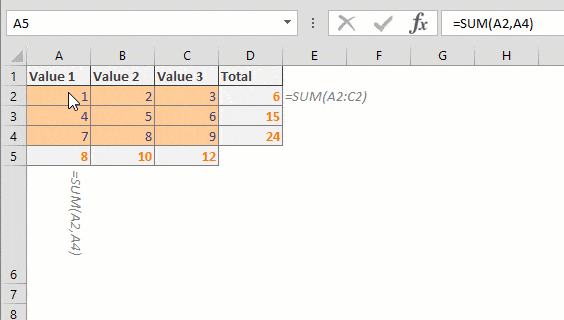A #REF! error in Excel indicates an invalid reference. This pesky error can pop up after removing a sheet, rows or columns, or copying a formula. In this guide, we're going to show you how to prevent a #REF error in Excel.

There are 3 common reasons that cause a #REF error in Excel:

1. Deleting a structural part like a sheet, row, or column that contain references used in a formula
2. Copying formulas that use relative references somewhere else where the references can't be populated
3. Formulas that generate invalid references

Let's take a look at these scenarios in more detail and what you can do to prevent a #REF error in Excel.

## #REF Error in Excel from deleting a row, a column, or a sheet

### Rows and columns

This is probably one of the most common scenarios that generate this error. Deleting a structural part in Excel may leave you with #REF! errors. This scenario happens when you are using a cell reference explicitly and the row or column is deleted.

For example, a cell contains =SUM(A2,B2,C2) formula. Deleting any of columns A, B or C causes the formula to return a #REF! error.If you see a #REF error in Excel after deleting a row or column, don't panic! First thing you need to do is undo the action. Simply press Ctrl + Z key combination to undo the deletion. This action will return the values back.

The next step is either removing the reference manually from the formula, or replacing individual references with range references. Excel can handle removing cells from named ranges automatically. Below are two examples to give you a better idea.

=SUM(A2,C2) – This is suitable if you are planning to remove column B

=SUM(A2:C2) – Use a range reference if you are planning to move more than one column.### Sheets

Unfortunately, you can't undo a deleting sheet action using Ctrl + Z. The only way to get your data back is to close the workbook without saving and then re-open it. After retrieving your deleted sheet, apply the necessary updates on the formulas. You may want to remove the part referencing that sheet or move the necessary data from the sheet you want to delete.

## #REF Error in Excel caused by copying and pasting cells

Another very frequent scenario - You may see a #REF error in Excel after copying and pasting a cell with a formula that is using relative references. The reason is that Excel updates relative references based on the cell where the formula is pasted.

For example, let's say you have a formula like =SUM(A2:C4) in cell F9. If you copy this cell and paste it into cell F2, you will see a #REF! error. In this case, Excel thinks that you moved the formula 7 columns above, and the references should be updated as well. However, there obviously are no negative references like A-5:C-3 in Excel.To prevent #REF! errors caused by copying and pasting cells, convert relative references into absolute ones. Excel does not update an absolute reference, no matter what.

Relative: =SUM(A2:C4)

Absolute: =SUM(\$A\$2:\$C\$4)

## Formulas generating invalid references

This scenario is less common than the first two. Some functions can point out or generate a reference in Excel. If you enter unsupported values into arguments, you may get a #REF! error. When you see a #REF! error in Excel, and the formula is complete with the required arguments, check out each argument one by one. Let's see some examples.

=VLOOKUP(1,A1:C4,5,0)

This VLOOKUP function tries to return a value from the 5th column of a 3-column range. Essentially, we get a #REF! error, because the range does not have a 5th column.

=INDEX(A1:C4,10,20)

Here, the INDEX function tries to return a value from the intersection of the 10th row and 20th column. However, these values exceed the supplied reference, A1:C4.

=OFFSET(A1,-1,-2)

We see an OFFSET function that references a cell located in negative references. Because this does not make sense conceptually, the formula returns a #REF! error.

=INDIRECT("invalid reference")

INDIRECT is a special function that can convert a text value into a reference. If you supply a string that can not be a reference, you will get a #REF error in Excel.